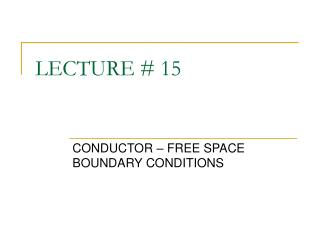DownloadDownload PresentationLECTURE # 15

# LECTURE # 15

Télécharger la présentation## LECTURE # 15

- - - - - - - - - - - - - - - - - - - - - - - - - - - E N D - - - - - - - - - - - - - - - - - - - - - - - - - - -
##### Presentation Transcript

1. LECTURE # 15 CONDUCTOR – FREE SPACE BOUNDARY CONDITIONS

2. Introduction + + + + + + + + free space conductor - - - - - - - -

4. OBJECTIVES • To relate mathematically fields that propagates between various materials. • To derive boundary conditions at the interface.

5. GRAPHICAL ILLUSTRATION E1n E1t E1 Medium # 1 Medium # 2 E2 E2n E2t E1 E2 Normal component E1t + E1n E2n + E2t Tangential component

6. NORMAL COMPONENTS E1n E1n E2n 1 S Maxwell’s equation (Gauss’s law) rs # 1 + + + + 0 h # 2 2 3 Assume h → 0 E2n Boundary condition for normal components

7. E1t E2t TANGENTIAL COMPONENTS E1t 1 2 # 1 Maxwell’s equation (Conservation of energy) h # 2 4 3 ℓ E2t 0 0 Assume again h → 0 Boundary condition for tangential components

8. CONDUCTOR – FREE SPACE BOUNDARY CONDITION # 1 Conductor Free space # 2 e0 e0 Boundary condition for normal components 0 Boundary conditions for conductor – free space/dielectric Boundary condition for tangential components 0

9. GRAPHICAL ILLUSTRATION Conductor Free space + + + Unit vector normal to the surface + + + + + rs

10. SUMMARIZED THE PRINCIPLES WHICH APPLY TO CONDUCTORS IN ELECTROSTATIC FIELDS • The static electric field intensity inside a conductor is zero. • The static electric field at the surface of a conductor is everywhere directed normal to that surface. • The conductor surface is an equipotential surface.

11. EXAMPLE 15.1 • Let potential field V = 100(x2 - y2) and point P( 2, -1, 3) lies on a conductor-free space boundary. • Determine the profile of the conductor. • Determine the electric field intensity at point P. • Determine the surface charge at point P.

12. EXAMPLE 15.2 • A potential field is given as V = (100e-5x sin 3y cos 4z) V. Let point P(0.1, p/12, p/24) be located at a conductor-free space boundary. At point P, find the magnitude of: (i) V; (ii) E; (iii) En; (iv) Et; (v) rs. Answer: 37.1 V, 233 V/m, 233 V/m, 0, 2.06 nC/m2

13. THANK YOU QUESTIONS AND ANSWERS